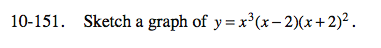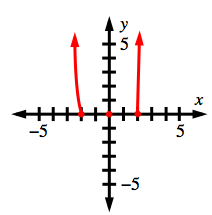Home > CCA2 > Chapter 10 > Lesson 10.3.1 > Problem10-151

10-151.

Sketch a graph of y = x3(x − 2)(x + 2)2. Homework Help ✎What are the x- and y-intercepts?
How does the function behave at each x-intercept?

What is the end behavior of the function?